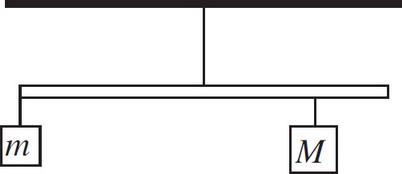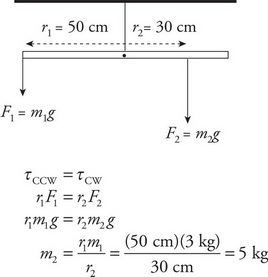# AP Physics 1 Question 236: Answer and Explanation

### Test Information

Question: 2366. A uniform meter stick of mass 1 kg is hanging from a thread attached at the stick's midpoint. One block of mass m = 3 kg hangs from the left end of the stick, and another block, of unknown mass M, hangs below the 80 cm mark on the meter stick. If the stick remains at rest in the horizontal position shown above, what is M ?

• A. 4 kg
• B. 5 kg
• C. 6 kg
• D. 8 kg